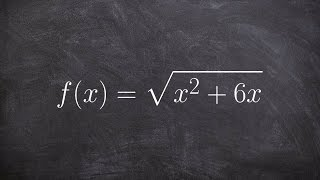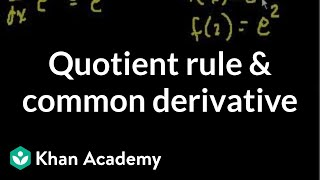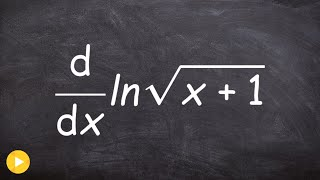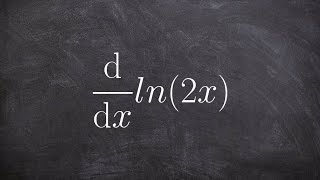Try NerdPal: our new app on iOS and Android !

Related Videos

Find the derivative of $\tan\left(x+1\right)$

Go!
Go!
1
2
3
4
5
6
7
8
9
0
a
b
c
d
f
g
m
n
u
v
w
x
y
z
.
(◻)
+
-
×
◻/◻
/
÷
2

e
π
ln
log
log
lim
d/dx
Dx
|◻|
θ
=
>
<
>=
<=
sin
cos
tan
cot
sec
csc

asin
acos
atan
acot
asec
acsc

sinh
cosh
tanh
coth
sech
csch

asinh
acosh
atanh
acoth
asech
acsch

VideosCalculus: Derivatives 2 | Taking derivatives | Differential Calculus | Khan AcademyCalculus - Find the derivative of the inverse of a radical equation, f(x)=sqrt(x^2 +6x); find g'(4)Quotient rule and common derivatives | Taking derivatives | Differential Calculus | Khan AcademyLocal linearization | Derivative applications | Differential Calculus | Khan AcademyCalculus - Using power rule with square root to take derivative on a logarithm, d(ln(sqrt(x+1)))/dxSnapXam A2

beta Got another answer? Verify it!

Go!
1
2
3
4
5
6
7
8
9
0
a
b
c
d
f
g
m
n
u
v
w
x
y
z
.
(◻)
+
-
×
◻/◻
/
÷
2

e
π
ln
log
log
lim
d/dx
Dx
|◻|
θ
=
>
<
>=
<=
sin
cos
tan
cot
sec
csc

asin
acos
atan
acot
asec
acsc

sinh
cosh
tanh
coth
sech
csch

asinh
acosh
atanh
acoth
asech
acsch

$\frac{d}{dx}\left(\tan\left(x+1\right)\right)$